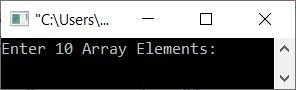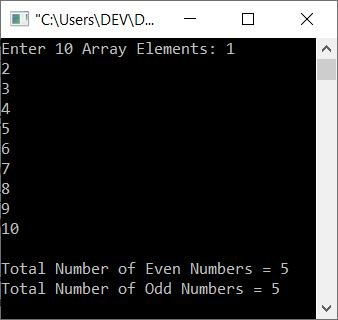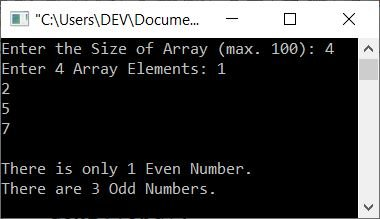# C++ Program to Count Even and Odd Numbers in an Array

This article provides you with some programs in C++ that count the number of even and odd numbers available in an array. The array must be entered by the user at run-time.

## Count the even and odd numbers in a 10-number array

The question is: write a program in C++ that receives an array of 10 numbers and counts the even and odd numbers available in the given array. The program given below is the answer to this question:

```#include<iostream>

using namespace std;
int main()
{
int arr, eve=0, odd=0, i;
cout<<"Enter 10 Array Elements: ";
for(i=0; i<10; i++)
cin>>arr[i];
for(i=0; i<10; i++)
{
if(arr[i]%2==0)
eve++;
else
odd++;
}
cout<<"\nTotal Number of Even Numbers = "<<eve;
cout<<"\nTotal Number of Odd Numbers = "<<odd;
cout<<endl;
return 0;
}```

The snapshot given below shows the initial output produced by the above C++ program on counting the total number of even and odd numbers in a given array:Now supply the input, say 1, 2, 3, 4, 5, 6, 7, 8, 9, 10 as ten array elements, and press the ENTER key to find and print the number of odd and even numbers like shown in the snapshot given below:The dry run of the above program goes like this:

• If the same ten elements are entered as in the previous sample run, each number is stored in the array one by one in the order arr = 1, arr = 2,... arr = 10.
• Since the user has now been given all ten numbers, the second for loop, which counts even and odd numbers, can start.
• That is, first i = 0 gets executed, which means 0 gets initialized to i. The initialization part executes only once.
• After initialization, the condition i<10 or 0<10 evaluates to be true.
• Therefore, program flow goes inside the loop.
• Inside the loop, the condition of if gets evaluated
• That is, the condition arr[i]%2==0 or arr%2==0 or 1%2==0 evaluates to be false.
• Therefore, program flow does not go inside if's body, but rather it goes to else's body and executes the statement `odd++;`
• Since the initial value of odd is 0, odd is now 1.
• Now the program flow goes to the update part of the loop, which is the increment part.
• So the value of i gets incremented; i = 1 now.
• Once again, the condition i<10 or 1<10 evaluates to true.
• Therefore, program flow again goes inside the loop.
• Again, the condition of if gets evaluated
• But this time, the condition arr[i]%2==0 or arr%2==0 or 2%2==0 or 0==0 evaluates to be true.
• Therefore, program flow goes inside its body (if's body), and this time the value of the eve variable gets incremented. As a result, eve=1 now.
• Again, the value of i gets incremented. So i is now equal to 2.
• And the condition i<10 or 2<10 evaluates to true once more.
• So, the program flow goes back into the loop, and this keeps happening until the condition is no longer true.
• In this way, the two variables, one for even number counting, and the other for odd number counting, hold the result.
• Therefore, just print the value of these two variables as a result, as shown in the sample run given above.

## Count the number of even and odd numbers in an array of n numbers

This is the modified version of the previous program because this program allows the user to define the size of the array along with its elements.

```#include<iostream>

using namespace std;
int main()
{
int tot, arr, i, eve=0, odd=0;
cout<<"Enter the Size of Array (max. 100): ";
cin>>tot;
cout<<"Enter "<<tot<<" Array Elements: ";
for(i=0; i<tot; i++)
cin>>arr[i];
for(i=0; i<tot; i++)
{
if(arr[i]%2==0)
eve++;
else
odd++;
}
cout<<endl;
if(eve>1)
cout<<"There are "<<eve<<" Even Numbers.";
else
{
if(eve==1)
cout<<"There is only 1 Even Number.";
else
cout<<"There is no any Even Number.";
}
cout<<endl;
if(odd>1)
cout<<"There are "<<odd<<" Odd Numbers.";
else
{
if(odd==1)
cout<<"There is only 1 Odd Number.";
else
cout<<"There is no any Odd Number.";
}
cout<<endl;
return 0;
}```

Here is its sample run with user input, 4 as size, and 1, 2, 5, and 7 as four array elements:C++ Quiz

« Previous Program Next Program »